## Bertrand's Postulate

If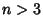, there is always at least one Prime betweenand. Equivalently, if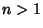, then there is always at least one Prime betweenand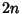. It was proved in 1850-51 by Chebyshev, and is therefore sometimes known as Chebyshev's Theorem. An elegant proof was later given by Erdös. An extension of this result is that if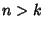, then there is a number containing a Prime divisorin the sequence,, ...,. (The casethen corresponds to Bertrand's postulate.) This was first proved by Sylvester, independently by Schur, and a simple proof was given by Erdös.

A related problem is to find the least value of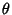so that there exists at least one Prime betweenandfor sufficiently large(Berndt 1994). The smallest known value is(Lou and Yao 1992).

References

Berndt, B. C. Ramanujan's Notebooks, Part IV. New York: Springer-Verlag, p. 135, 1994.

Erdös, P. Ramanujan and I.'' In Proceedings of the International Ramanujan Centenary Conference held at Anna University, Madras, Dec. 21, 1987. (Ed. K. Alladi). New York: Springer-Verlag, pp. 1-20, 1989.

Lou, S. and Yau, Q. A Chebyshev's Type of Prime Number Theorem in a Short Interval (II).'' Hardy-Ramanujan J. 15, 1-33, 1992.